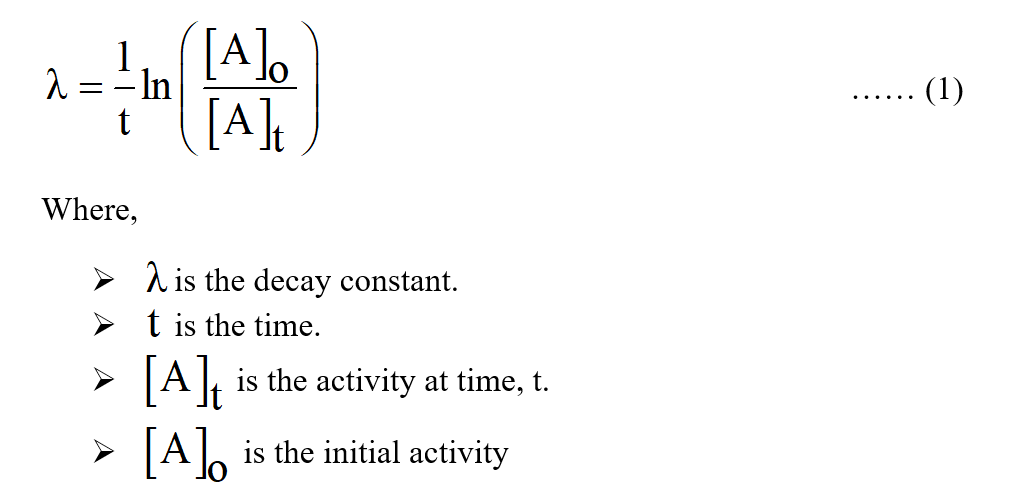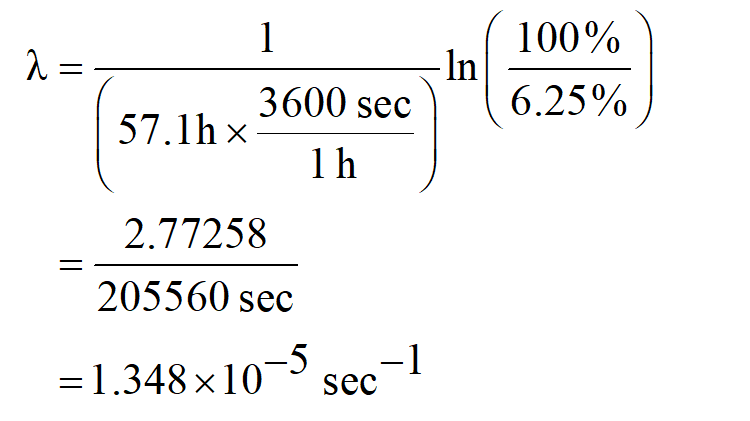# What is the half‑life of an isotope that decays to 6.25%6.25% of its original activity in 57.1 h?

Question
36 views

What is the half‑life of an isotope that decays to 6.25%6.25% of its original activity in 57.1 h?

check_circle

Step 1

The half-life of the isotopes can be described as the time at which the amount of the activity becomes half of the original activity.

Step 2

Consider the initial activity is 100 %. At time 57.1 h, the activity remain is 6.25 % of its original activity.

The expression to calculate the decay constant is shown below:Step 3

The value of time is 57.1 h.

The value of the initial activity is 100 %.

The value of the activity at the time is 6.25 %.

Substitute the value in equation (1).Therefore, the value of the decay constant is 1.348×10-5 sec-1.

...

### Want to see the full answer?

See Solution

#### Want to see this answer and more?

Solutions are written by subject experts who are available 24/7. Questions are typically answered within 1 hour.*

See Solution
*Response times may vary by subject and question.
Tagged in